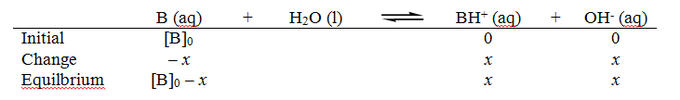## Base Dissociation Constant

#### Learning Objective

• Calculate the Kw (water dissociation constant) using the following equation: Kw = [H+] x [OH−]  and manpulate the formula to determine [OH−] = Kw/[H+] or [H+]=Kw/[OH-]

#### Key Points

• The base dissociation constant KbE measures a base’s basicity, or strength.
• Kb is related to the acid dissociation constant, Ka, by the simple relationship pKa + pKb = 14, where pKb and pKa are the negative logarithms of Kb and Ka, respectively.
• Kb and Ka are also related through the ion constant for water, Kw, by the relationship $K_W=K_a\times K_b$ .

#### Term

• conjugate acidthe species created when a base accepts a proton

In chemistry, a base is a substance that can accept hydrogen ions (protons) or, more generally, donate a pair of valence electrons. The base dissociation constant, Kb, is a measure of basicity—the base’s general strength. It is related to the acid dissociation constant, Ka, by the simple relationship pKa + pKb = 14, where pKb and pKa are the negative logarithms of Kb and Ka, respectively. The base dissociation constant can be expressed as follows:

$K_b = \dfrac{[\text{BH}^+][\text{OH}^-]}{\text{B}}$

where $\text{B}$ is the base, $\text{BH}^+$ is its conjugate acid, and $\text{OH}^-$ is hydroxide ions.

## The Base Dissociation Constant

Historically, the equilibrium constant Kb for a base has been defined as the association constant for protonation of the base, B, to form the conjugate acid, HB+.

$B(aq) + H_2O(l) \leftrightharpoons HB^+(aq) + OH^-(aq)$

As with any equilibrium constant for a reversible reaction, the expression for Kb takes the following form:

$K_{b} = \frac{[OH^{-}][HB^{+}]}{[B]}$

Kb is related to Ka for the conjugate acid. Recall that in water, the concentration of the hydroxide ion, [OH], is related to the concentration of the hydrogen ion by the autoionization constant of water:

$K_W=[H^+][OH^-]$

Rearranging, we have:

$[OH^{-}] = \frac{K_{w}}{[H^{+}]}$

Substituting this expression for [OH] into the expression for Kb yields:

$K_{b} = \frac{K_{w}[HB^{+}]}{[B][H^{+}]} = \frac{K_{w}}{K_{a}}$

Therefore, for any base/conjugate acid pair, the following relationship always holds true:

$K_W=K_aK_b$

Taking the negative log of both sides yields the following useful equation:

$pK_a+pK_b=14$

In actuality, there is no need to define pKb separately from pKa, but it is done here because pKb values are found in some of the older chemistry literature.

## Calculating the pH of a Weak Base in Aqueous Solution

The pH of a weak base in aqueous solution depends on the strength of the base (given by Kb) and the concentration of the base (the molarity, or moles of the base per liter of solution). A convenient way to find the pH for a weak base in solution is to use an ICE table: ICE stands for “Initial,” “Change,” and”Equilibrium.”

Before the reaction starts, the base, B, is present in its initial concentration [B]0, and the concentration of the products is zero. As the reaction reaches equilibrium, the base concentration decreases by x amount; given the reaction’s stoichiometry, the two products increase by x amount. At equilibrium, the base’s concentration is [B]0x, and the two products’ concentration is x.ICE diagramAn ICE diagram for a weak base in aqueous solution.

The Kb for the reaction is:

$K_{b} = \frac{[BH^+][OH^-]}{[B]}$

Filling in the values from the equilibrium line gives:

$K_{b} = \frac{x^2}{[B]_{0}-x}$

This quadratic equation can be solved for x. However, if the base is weak, then we can assume that x will be insignificant compared to [B]0, and the approximation [B]0x ≈ [B]0 can be used. The equation simplifies to:

$K_{b} = \frac{x^2}{[B]_{0}}$

Since x = [OH], we can calculate pOH using the equation pOH = –log[OH]; we can find the pH using the equation 14 – pOH = pH.